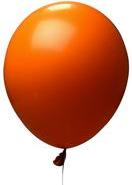# Using Statistical Regression Methods in Education Research# 5.11 Conclusions

In this module we have looked at regression models that can be applied when our outcome is represented by an ordinal variable. Several authors (e.g., Ananth & Kleinbaum, 1997) argue that ordinal models are under-used in social sciences, with researchers often reducing ordinal data to a series of binary logistic regressions. In contrast ordinal regression models take advantage of the ordinality in the outcome by summarising the relationships between explanatory variables and the outcome in a single parsimonious model. However the most popular approach to ordinal regression - the proportional odds (PO) model - makes an substantial simplifying assumption that the ORs associated with each explanatory variable are the same over the cumulative splits in the outcome. This assumption has to be tested if ordinal models are to be applied appropriately. We have noted that the statistical test of the PO assumption is over-conservative and the PO assumption is frequently rejected particularly when the number of explanatory variables is large (Brant, 1990), the sample size is large (Allison, 1999; Clogg & Shihadeh, 1994) or there is a continuous explanatory variable in the model (Allison, 1999). Therefore we have seen how to evaluate the ordinal PO model by completing a series of binary logistic regressions at each of the cumulative splits in the data, and how this can allow us to directly evaluate the consistency in ORs across an ordinal outcome. Where the PO assumption is justified ordinal regression models can be a powerful means of summarising relationships that utilises all the information present in the ordinal outcome. As you can tell, there is some debate by statisticians about when to use Ordinal Regression. A sure sign that you have reached the end of a tricky Module... Well done!## Take the Ordinal Regression Quiz###### Check your understanding of logistic regression.Now you can take a look at the quiz and the exercise if you like!Sadly we cannot provide any tangible reward for your endeavours so we have added these three pictures of balloons as a token gesture. We hope that you will find your new found quantitative research skills useful!

 HomeModulesSite GuideModule 5 ContentsResources